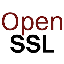OpenSSL "rsautl -encrypt -raw" - Data Too Large Error

Q

Why am I getting the "data too large for key size" error with OpenSSL "rsautl -encrypt -raw" command? My input data is the same size as the RSA key and I am using no padding.

✍: FYIcenter.com

AIn most case, you should be able to use the OpenSSL "rsautl -encrypt -raw" command to encrypt input data of the same size as the RSA public key.

But sometimes, you will get the "data too large for key size" error, if the integer value represented by the input data is larger than the modulus value of the RSA public key.

For example, if the input data is a 128-byte of 0xFF, its integer value will be larger than the modulus value of any 128-byte (1024-bit) RSA public keys.

```C:\Users\fyicenter>dir 128-byte-ff.txt
128 128-byte-ff.txt

C:\Users\fyicenter>bin2hex 128-byte-ff.txt output.hex

C:\Users\fyicenter>type output.hex
ffffffffffffffffffffffffffffffffffffffffffffffffffffffffffffffff
ffffffffffffffffffffffffffffffffffffffffffffffffffffffffffffffff
ffffffffffffffffffffffffffffffffffffffffffffffffffffffffffffffff
ffffffffffffffffffffffffffffffffffffffffffffffffffffffffffffffff

C:\Users\fyicenter>\local\openssl\openssl.exe

OpenSSL> pkey -pubin -in my_rsa_pub.key -text -noout
Public-Key: (1024 bit)
Modulus:
00:a1:1e:80:d3:d1:a9:bc:80:27:00:b5:92:79:87:
4e:62:42:3c:89:da:6e:a3:ea:93:5e:f1:7c:0b:db:
32:bd:5d:ef:d5:73:95:5c:db:e0:cd:26:c3:4a:6b:
b8:13:e6:6a:8e:8c:d8:f7:22:95:22:d2:2a:3c:1f:
d2:6e:43:18:ec:e8:df:36:79:b1:22:4f:ee:c8:3e:
b1:f2:b3:80:f9:ab:ab:d6:7c:30:62:c2:e8:86:cf:
38:e2:43:1c:0f:99:15:70:80:8d:22:e9:b8:57:d7:
80:2e:29:8e:7c:e0:2f:9e:b7
Exponent: 65537 (0x10001)

OpenSSL> rsautl -encrypt -pubin -inkey my_rsa_pub.key -in 128-byte-ff.txt
-out cipher.txt -raw
RSA operation error
7000:error:04068084:rsa routines:RSA_EAY_PUBLIC_ENCRYPT:data too large for modulus
:.\crypto\rsa\rsa_eay.c:221:error in rsautl
```

The output tells us that:

• The input data is 128-byte long, which is the same as the RSA public key.
• The integer value of the input data is 0xffffffff...ffff", the largest integer that can stored in 128 bytes.
• The modulus value of the RSA public key is 0xa11e80d3...9eb7", which is smaller than the integer value of the input data. Note that the first byte of 0x00 in the modulus output is not part of the modulus.
• OpenSSL "rsautl -encrypt -raw" command returns the "data too large for modulus" error.

2017-04-22, 4493👍, 0💬